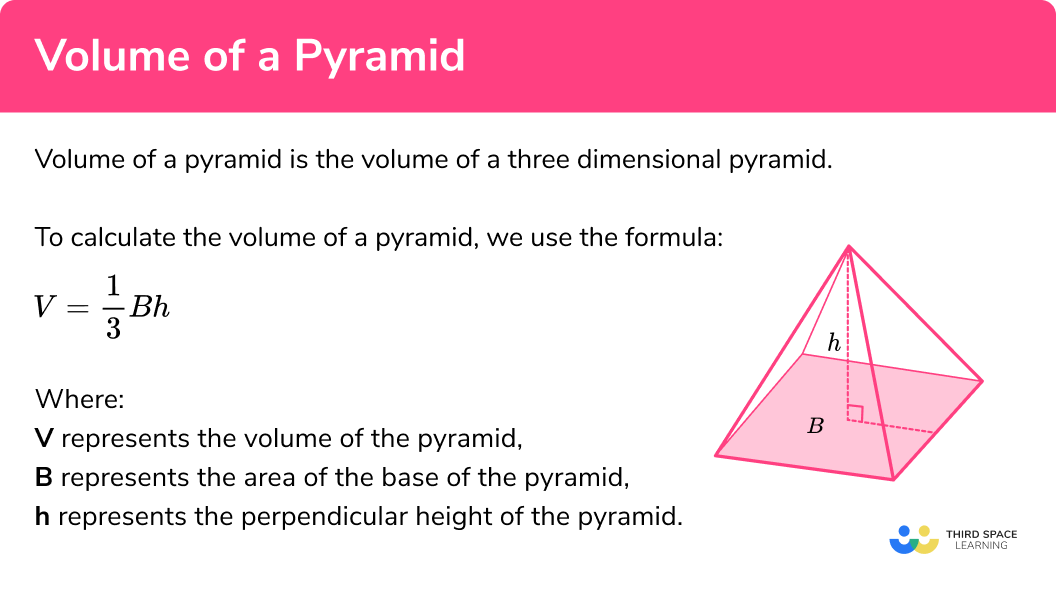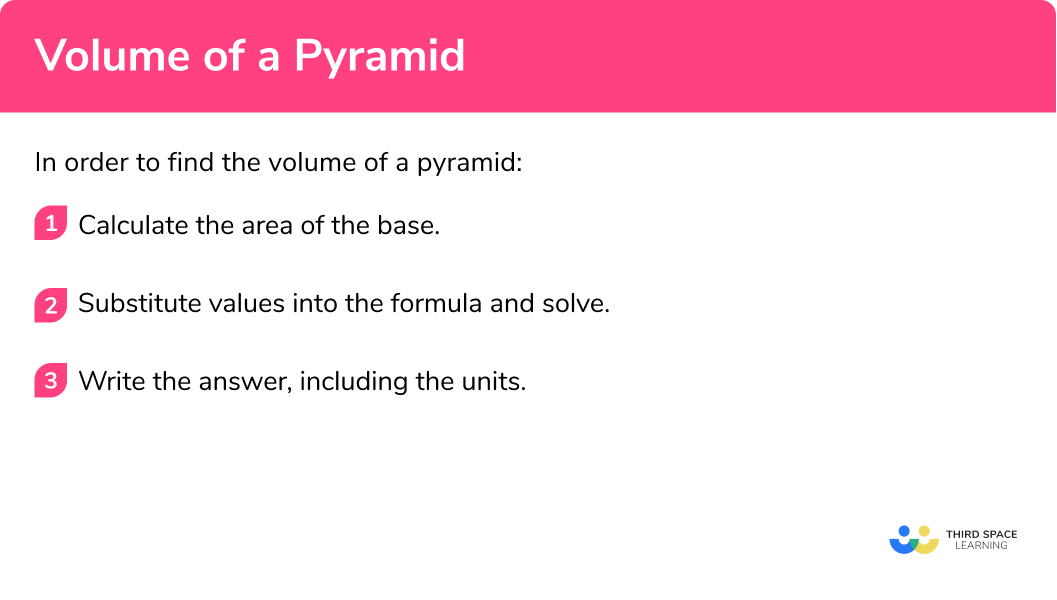# Volume Of A Pyramid

Here we will learn about the volume of a pyramid, including how to find the volume of a pyramid using the base.

There are also volume of a pyramid worksheets based on Edexcel, AQA and OCR exam questions, along with further guidance on where to go next if you’re still stuck.

## What is the volume of a pyramid?

The volume of a pyramid is how much space there is inside a pyramid.

For example, the great pyramids of Giza have an approximate volume of 2600000m^3 .

To calculate the volume of a pyramid, we need to know certain lengths of the pyramid.

A pyramid is a three dimensional shape made up of flat faces.  It has a base and triangular faces which meet at a point, called the apex.  The vertical height is the length from the base to the apex and is perpendicular to the base of the pyramid. he pyramid could have a square base, a triangular base, it could be a pentagonal pyramid or a hexagonal pyramid. The volume formula is the same no matter what the shape of the base.

To calculate the volume of a pyramid, we use the formula:

V=\frac{1}{3}Bh

where:

• V represents the volume of the pyramid,
• B represents the area of the base of the pyramid,
• h represents the perpendicular height of the pyramid.

This formula can be applied to any pyramid where the base is a polygon. It can also be used to find the volume of a cone.

E.g. calculate the volume of a square-based pyramid where the base has a side length of 4 \ cm and the height of the pyramid is 6 \ cm.

\begin{aligned} V&=\frac{1}{3}\times{B}\times{h}\\\\ &=\frac{1}{3}\times(4\times{4})\times{6}\\\\ &=32\text{ cm}^{3} \end{aligned}

Note:

The volume of any pyramid is one third of the volume of a prism with the same base shape and height h.

### What is the volume of a pyramid?## How to calculate the volume of a pyramid

In order to calculate the volume of a pyramid:

1. Calculate the area of the base.
2. Substitute values into the formula and solve.
3. Write the answer, including the units.

### How to calculate the volume of a pyramid## Volume of a pyramid examples

### Example 1: calculating the volume with a diagram included

Calculate the volume of the pyramid below.

1. Calculate the area of the base.

The base is a square with side length 7 \ cm .

\text{Area of base}=7\times 7=49

2Substitute values into the formula and solve.

As B=49cm^2 and h=9cm , substituting these into the formula for the volume of a pyramid, we get:

\begin{aligned} V&=\frac{1}{3}\times{B}\times{h}\\\\ &=\frac{1}{3}\times{49}\times{9}\\\\ &=147 \end{aligned}

3Write the answer, including the units.

V=147\text{ cm}^{3}

### Example 2: rectangular based pyramid

Calculate the volume of this pyramid. Write your answer to 1 decimal place.

Calculate the area of the base.

Substitute values into the formula and solve.

Write the answer, including the units.

### Example 3: without a diagram

Calculate the volume of a square-based pyramid where the side length of the base is 8 \ m and perpendicular height is 12 \ m .

Calculate the area of the base.

Substitute values into the formula and solve.

Write the answer, including the units.

### Example 4: without a diagram

Find the volume of a square-based pyramid with the side length of the base equal to 12.3 \ mm , and the height of the pyramid equal to 18.2 \ mm .  Give your answer correct to 2 decimal places.

Calculate the area of the base.

Substitute values into the formula and solve.

Write the answer, including the units.

### Example 5: rectangular base

ABCDE is a rectangular base pyramid. The point F is the centre of the base, directly below the vertex E , and G is the midpoint of the line AD . Given that EF=6cm and DG=2.5cm, calculate the volume of this pyramid.

Calculate the area of the base.

Substitute values into the formula and solve.

Write the answer, including the units.

### Example 6: calculating the height

Calculate the height of a pyramid with volume 40 \ cm^3 and base area 12 \ cm^3 .

Calculate the area of the base.

Substitute values into the formula and solve.

Write the answer, including the units.

### Common misconceptions

• The height is the perpendicular height

The height of the pyramid needs to be the perpendicular height not the slant height.  This is the height that is at a right-angle to the base. You may need to find this height by using trigonometry or Pythagoras’ Theorem.

• Volume has cubic units

The volume will have cube units such as cubic centimetres ( cm^3 ) or cubic metres ( m^3 ).

• Be accurate

When there are two or more steps in your workings, do not round your workings.  For example – do not round the area of the base.  Only round at the end of the question so that your answer is accurate.

• Take care with rounding

At the end of the question, make sure you round your answer to the correct number of decimal places or significant figures.

Volume of a pyramid is part of our series of lessons to support revision on pyramids. You may find it helpful to start with the main pyramid lesson for a summary of what to expect, or use the step by step guides below for further detail on individual topics. Other lessons in this series include:

### Practice volume of a pyramid questions

1. Calculate the volume of the pyramid below.45 \text{ cm}^315 \text{ cm}^311 \text{ cm}^330 \text{ cm}^3\text{Area of base}=3\times{3} =9

As B=9cm^2 and h=5cm ,

\begin{aligned} V&=\frac{1}{3}\times{b}\times{h} \\\\ &=\frac{1}{3}\times{9}\times{5} \\\\ &=15 \end{aligned}

V=15\text{ cm}^{3}

2. Find the volume. Give your answer correct to 1 decimal place:229.5 \text{ mm}^3668.8 \text{ mm}^3229.6 \text{ mm}^3229.6 \text{ cm}^3\text{Area of base}=8.7\times{8.7} =75.69

As B=75.69mm^2 and h=9.1mm ,

\begin{aligned} V&=\frac{1}{3}\times{B}\times{h}\\\\ &=\frac{1}{3}\times{75.69}\times{9.1}\\\\ &=229.593 \end{aligned}

V=229.6\text{ mm}^{3}

3. Find the volume of a square-based pyramid where the side length of the base is 6 \ m and the height is 10 \ m.

300 \text{ m}^3220 \text{ m}^3360 \text{ m}^3120 \text{ m}^3\text{Area of base}=6\times{6} =36

As B=36m^2 and h=10m ,

\begin{aligned} V&=\frac{1}{3}\times{B}\times{h}\\\\ &=\frac{1}{3}\times{36}\times{10}\\\\ &=120 \end{aligned}

V=120\text{ m}^{3}

4. ABCDE is a square based pyramid. ABCD is the base of the pyramid. E is the apex, directly above the centre of the base.

Given that AB=3.5cm and h=7.2cm, calculate the volume of the pyramid.

16.3 \text{ cm}^333.6 \text{ cm}^329.4 \text{ cm}^3444.1 \text{ cm}^3\text{Area of base}=3.5\times{3.5} =12.25

As B=12.25cm^2 and h=7.2cm ,

\begin{aligned} V&=\frac{1}{3}\times{B}\times{h}\\\\ &=\frac{1}{3}\times{12.25}\times{7.2}\\\\ &=29.4 \end{aligned}

V=29.4\text{ cm}^{3}

5. Calculate the volume of this pyramid:35 \text{ cm}^325 \text{ cm}^3105 \text{ cm}^349 \text{ cm}^3\text{Area of base}=7\times{5} =35

As B=35cm^2 and h=3cm ,

\begin{aligned} V&=\frac{1}{3}\times{B}\times{h}\\\\ &=\frac{1}{3}\times{35}\times{3}\\\\ &=35 \end{aligned}

V=35\text{ cm}^{3}

6. Find the height of a pyramid with volume 56 \ cm^3 and base area 21 \ cm^2.

8 \text{ cm}7 \text{ cm}2.7 \text{ cm} \ (1dp)0.\dot{8}\text{ cm}As V=56cm^3 and B=21cm^2,

\begin{aligned} V&=\frac{1}{3}\times{B}\times{h}\\\\ 56&=\frac{1}{3}\times{21}\times{h}\\\\ 56&=7h\\\\ &h=8 \end{aligned}

h=8\text{ cm}

### Volume of a pyramid GCSE questions

1. A square-based pyramid has a base with side length 12 \ cm.

The perpendicular height of the pyramid is 17 \ cm.

Calculate the volume of the pyramid. State the units of your answer.

(3 marks)

\frac{1}{3}\times 12^2 \times 17

(1)

816

(1)

\text{cm}^{3}

(1)

2. A pyramid has the volume 460 \ cm^3 and a base with the area 200 \ cm^2.

Calculate the height of the pyramid.

(1 mark)

C \ – \ 6.9 \ cm

(1)

3. A solid is made from a pyramid on top of a cuboid.Calculate the volume of the compound 3D shape.

(3 marks)

6\times 5\times 4=120

(1)

\frac{1}{3}\times (6\times 5) \times 3=30

(1)

120+30=150

(1)

## Learning checklist

You have now learned how to:

• Calculate volumes of pyramids

## Still stuck?

Prepare your KS4 students for maths GCSEs success with Third Space Learning. Weekly online one to one GCSE maths revision lessons delivered by expert maths tutors.

Find out more about our GCSE maths tuition programme.